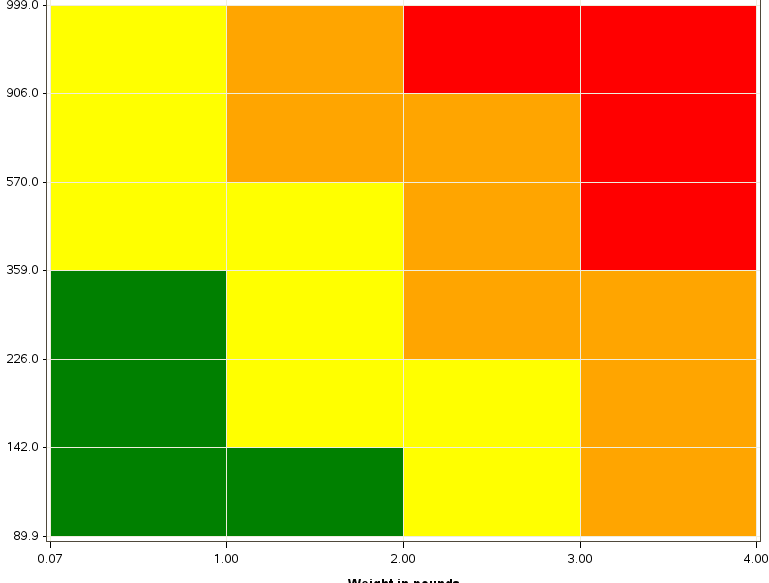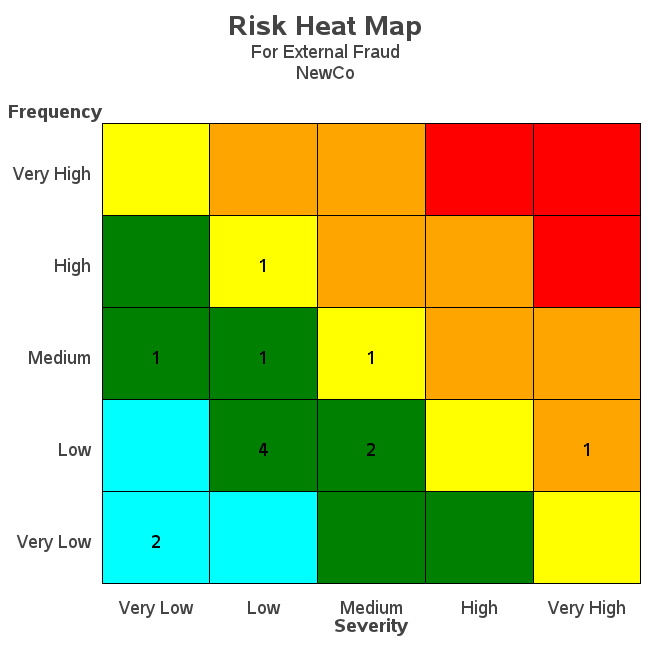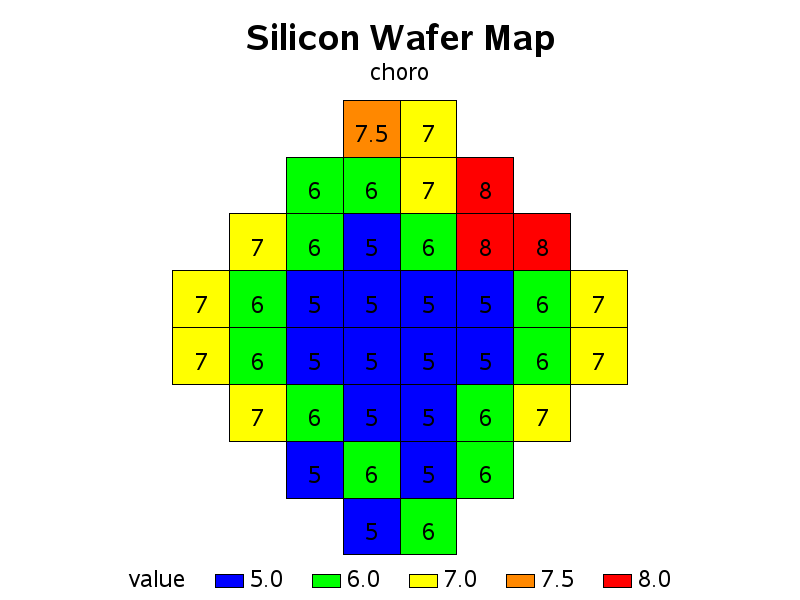## Data for graph

Good day for all!

I'm working in Guide and I want to create a graph like this (with different numbers, I have problem with numbers for graph not with graph):I'm drawing blocks with annotation help. There is my graph.

goptions reset=all cback=white border htitle=12pt htext=10pt;

data anno;

length function color \$64;

retain line 0 xsys ysys "2" ;

set TESTLIB.TESTFORROSK4;

function="move"; x=0.07; y=89.895184; output;

function="bar";  x=1; y=142; color="green"; line=1; style="solid"; output;

function="move"; x=1; y=89.895184; output;

function="bar";  x=2; y=142; color="green"; line=1; style="solid"; output;

function="move"; x=2; y=89.895184; output;

function="bar";  x=3; y=142; color="yellow"; line=1; style="solid"; output;

function="move"; x=3; y=89.895184; output;

function="bar";  x=4; y=142; color="orange"; line=1; style="solid"; output;

function="move"; x=0.07; y=142; output;

function="bar";  x=1; y=226; color="green"; line=1; style="solid"; output;

function="move"; x=1; y=142; output;

function="bar";  x=2; y=226; color="yellow"; line=1; style="solid"; output;

function="move"; x=2; y=142; output;

function="bar";  x=3; y=226; color="yellow"; line=1; style="solid"; output;

function="move"; x=3; y=142; output;

function="bar";  x=4; y=226; color="orange"; line=1; style="solid"; output;

function="move"; x=0.07; y=226; output;

function="bar";  x=1; y=359; color="green"; line=1; style="solid"; output;

function="move"; x=1; y=226; output;

function="bar";  x=2; y=359; color="yellow"; line=1; style="solid"; output;

function="move"; x=2; y=226; output;

function="bar";  x=3; y=359; color="orange"; line=1; style="solid"; output;

function="move"; x=3; y=226; output;

function="bar";  x=4; y=359; color="orange"; line=1; style="solid"; output;

function="move"; x=0.07; y=359; output;

function="bar";  x=1; y=570; color="yellow"; line=1; style="solid"; output;

function="move"; x=1; y=359; output;

function="bar";  x=2; y=570; color="yellow"; line=1; style="solid"; output;

function="move"; x=2; y=359; output;

function="bar";  x=3; y=570; color="orange"; line=1; style="solid"; output;

function="move"; x=3; y=359; output;

function="bar";  x=4; y=570; color="red"; line=1; style="solid"; output;

function="move"; x=0.07; y=570; output;

function="bar";  x=1; y=906; color="yellow"; line=1; style="solid"; output;

function="move"; x=1; y=570; output;

function="bar";  x=2; y=906; color="orange"; line=1; style="solid"; output;

function="move"; x=2; y=570; output;

function="bar";  x=3; y=906; color="orange"; line=1; style="solid"; output;

function="move"; x=3; y=570; output;

function="bar";  x=4; y=906; color="red"; line=1; style="solid"; output;

function="move"; x=0.07; y=906; output;

function="bar";  x=1; y=999; color="yellow"; line=1; style="solid"; output;

function="move"; x=1; y=906; output;

function="bar";  x=2; y=999; color="orange"; line=1; style="solid"; output;

function="move"; x=2; y=906; output;

function="bar";  x=3; y=999; color="red"; line=1; style="solid"; output;

function="move"; x=3; y=906; output;

function="bar";  x=4; y=999; color="red"; line=1; style="solid"; output;

run;

proc gplot data=TESTLIB.TESTFORROSK4;

plot D*B / haxis=axis1 vaxis=axis2

annotate=anno

GRID;

symbol1 interpol=none value=none color=white;

axis1  color= black ORDER=0.07 1 2 3 4 ;

axis2  color= black ORDER=89.895184 142 226 359 570 906 999  ;

run;

quit;

Now I need to put  these numbers in  coordinates automatically. Get them off in the previous table. My data from previous table:

 Y X 6.9320657556 . 13.864131511 . 27.728263022 . 55.456526045 . 110.91305209 . 221.82610418 . 443.65220836 . . 0.0211314174 . 0.0845256696 . 0.3381026786 . 0.3381026786 . 1.3524107143

I didn't know how I can accumulate numbers in one table. I try to adapt this algorithm to guide but I have some problem with it.

for (i=0; i<6; i++){

for (j=1; j<7; j++){

x = i, y = j;

}

}

For example, I need ability to return to the previouse numbers for "function=move".

There is what I'm wrote (the first steps):

DATA fullDotsTable;

SET WORK.MERGEABDOTS;

%let tempA;

if (_n_=1) then do;

call symput("tempA", A);

end;

else do;

x = B; y = A;

yv = symget("tempA");

call symput("tempA", A);

end;

RUN;

I think I need some SQL in my code but I don't know how I can succes with it. Please, help!

1 ACCEPTED SOLUTION

Accepted Solutions

## Re: Data for graph

Or, here's another one that might be more similar to what you're wanting...4 REPLIES 4

## Re: Data for graph

I think the following example might help you. I use Proc SQL to calculate the average x/y center of each square, and then annotate some text there...

proc sql noprint;

create table lablanno as

select unique id, avg(x) as x, avg(y) as y, value

from my_data

group by id;

quit; run;

data lablanno; set lablanno;

xsys='2'; ysys='2'; hsys='3'; when='A';

position='5'; size=4; style="albany amt";

function='label';

text=trim(left(value));

run;## Re: Data for graph

Thank you for the example! It's not exactly what I wanted but it will be very helpful for me anyway.

## Re: Data for graph

Or, here's another one that might be more similar to what you're wanting...